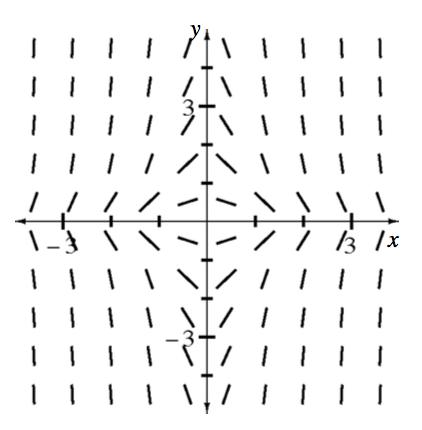### Home > APCALC > Chapter 12 > Lesson 12.1.3 > Problem12-35

12-35.

The diagram at right shows a slope field.

1. Explain why $\frac { d y } { d x }$ must be a function of both $x$ and $y$.

Are the slopes in the diagram parallel anywhere?

2. Sketch a solution curve that passes through the point $(0, 3)$.

Your curve should look like a bell-shaped curve with a maximum at $\left(0, 3\right)$ and a horizontal asymptote of $y = 0$.

3. The slope field is for the differential equation $\frac { d y } { d x }= -2xy$ . Write the equation of your solution curve.

$\int\frac{dy}{y}=-2\int x dx$

Once you integrate you should have a $'+ C$'. Use the point $(0, 3)$ to solve for the value of $C$.